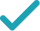Type a math problemFactorSolution Steps
Solution Steps
Factor out .
EvaluateGraphGiving is as easy as 1, 2, 3
Get 1,000 points to donate to a school of your choice when you join Give With Bing
x\left(2x+3\right)
Factor out x.
2x^{2}+3x=0
Quadratic polynomial can be factored using the transformation ax^{2}+bx+c=a\left(x-x_{1}\right)\left(x-x_{2}\right), where x_{1} and x_{2} are the solutions of the quadratic equation ax^{2}+bx+c=0.
x=\frac{-3±\sqrt{3^{2}}}{2\times 2}
All equations of the form ax^{2}+bx+c=0 can be solved using the quadratic formula: \frac{-b±\sqrt{b^{2}-4ac}}{2a}. The quadratic formula gives two solutions, one when ± is addition and one when it is subtraction.
x=\frac{-3±3}{2\times 2}
Take the square root of 3^{2}\approx 9.
x=\frac{-3±3}{4}
Multiply 2 times 2.
x=\frac{0}{4}
Now solve the equation x=\frac{-3±3}{4} when ± is plus. Add -3 to 3.
x=0
Divide 0 by 4.
x=\frac{-6}{4}
Now solve the equation x=\frac{-3±3}{4} when ± is minus. Subtract 3 from -3.
x=-\frac{3}{2}
Reduce the fraction \frac{-6}{4}=-1.5 to lowest terms by extracting and canceling out 2.
2x^{2}+3x=2x\left(x-\left(-\frac{3}{2}\right)\right)
Factor the original expression using ax^{2}+bx+c=a\left(x-x_{1}\right)\left(x-x_{2}\right). Substitute 0 for x_{1} and -\frac{3}{2}=-1.5 for x_{2}.
2x^{2}+3x=2x\left(x+\frac{3}{2}\right)
Simplify all the expressions of the form p-\left(-q\right) to p+q.
2x^{2}+3x=2x\times \left(\frac{2x+3}{2}\right)
Add \frac{3}{2}=1.5 to x by finding a common denominator and adding the numerators. Then reduce the fraction to lowest terms if possible.
2x^{2}+3x=x\left(2x+3\right)
Cancel out 2, the greatest common factor in 2 and 2.